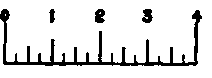When the object which is to be drawn is not so large but that it can be easily shown actual size (or full size as it is called) on a sheet of paper of convenient dimensions, it is good practice to draw the piece full size. In many cases, however, the machine, or the building, or whatever is to be drawn, is so large that it would be impossible to draw it full size. Then the drawing is made to some reduced scale, that is, all the dimensions are drawn smaller than the actual dimensions of the object itself; all dimensions being reduced in the same proportion. For example, if a piece is to be drawn ½ size, the distance from one point to another on the drawing would be ½ what it is on the piece itself; if the drawing is \ size, the distance on the drawing would be \ what it is on the piece itself, and so on. In dimensioning such a drawing, the dimension which is written on the drawing is the actual dimension of the piece, and not the distance which is measured on the drawing. This fact must be very clearly understood by the student.Fig. 29. Conventional Drawing of Thread in a Pipe Fitting.

## Methods Of Reducing Dimensions

The common method of reducing all the dimensions in the same proportion is to choose a certain distance and let that distance represent one foot, this distance is then divided into twelve parts and each one of these parts represents an inch; then if half and quarter inches are required these twelfths are subdivided into halves, quarters, etc., until the subdivisions become so small that they cannot be used. We now have a scale which represents the common foot rule with its subdivisions into inches and fractions; but our new foot is smaller than the ordinary distance which we call a foot, and of course its subdivisions are proportionately smaller. When we make a measurement on the drawing we make it with our reduced foot rule and when we make a measurement on the machine itself we make it with the common foot rule.

## Draftmen's Scales And Their Use

Draftsmen's scales can be bought which have different distances thus divided, so that if the draftsman wishes to draw a piece ¼ size he looks over his scale until he finds a distance of 3 inches (which is of course of a foot) divided as explained above, and he uses this to measure with on his drawing. His drawing would then be made to a scale of 3 inches to the foot. In the same way, if he wishes to make his drawing 1/12 size he finds on his scale 1 inch divided into twelfths and fractions of twelfths and uses this as his standard of measurement; if he wishes to make his drawing 1/48 size he uses a quarter inch with its subdivisions.

Sometimes if the piece to be drawn is too small to be satisfactorily shown full size, the drawing is made to an enlarged scale, such as twice size, three times size, etc.

The mistake of choosing the wrong distance to use on a scale is often made. For example, if one wishes to draw a piece ¼ size, he may look over his scale for a place marked i, and use this for his standard for ¼ size, which is wrong. The figure on the scale indicates the distance which is divided up to represent 1 foot, so that the part of the scale which has ¼ marked on it means that ¼ of an inch is divided up into twelfths, or in other words, if a drawing is made according to that scale it will be 1/48 size.Fig. 30. Reduced Scale Drawing. Actual Scale, 3"= 1'.

## Usual Scales For Drawings

The best scales for shop drawings in the United States are those readily derived from the common foot rule, such as full size, 6 inches = 1 foot, 3 inches = 1 foot, 1½ inches = 1 foot. These are the most common, most easily read from an ordinary scale, and one of these can usually be adopted. The student should learn to read these from an ordinary scale without being confined to a special graduation. To do this it is not necessary to divide each dimension by 2, 4, and 8 to get ½ size, ¼ size, or ⅛ size, and then lay down the result. For ½ size, or 6 inches = 1 foot, ½ inch on an ordinary rule represents 1 inch. Hence, each ½ inch may be read as 1 inch, and its subdivisions accordingly, thus:For 3 inches = 1 foot, or ¼ size, ¼ inch represents 1 inch, and looks thus:For 1½ inches = 1 foot, or ⅛ size, ⅛ inch represents 1 inch, and looks thus:It is very easy to get accustomed to this, and it saves much time and trouble hunting up a special scale every time.

The other allowable scales, less common, but sometimes necessary on large work, are 1 inch = 1 foot, ¾ inch = 1 foot, ½ inch = 1 foot, ⅜ inch = 1 foot, ¼ inch = 1 foot, and ⅛ inch = 1 foot. To use these scales conveniently, special graduation is desirable.

Every drawing should have the scale to which it is made plainly marked upon it, as a part of, or adjacent to, the title.

Fig. 30 shows a detail which has been reduced in making the cut so that its dimensions are on a scale of 3 inches per foot, or ¼ size. By applying his scale to this cut and comparing the readings of his scale with the dimensions as given, the student will gain a clear understanding of a reduced scale drawing. None of the other cuts in this book are reduced for the purpose of scaling, hence have no even relation to their dimensions as given.